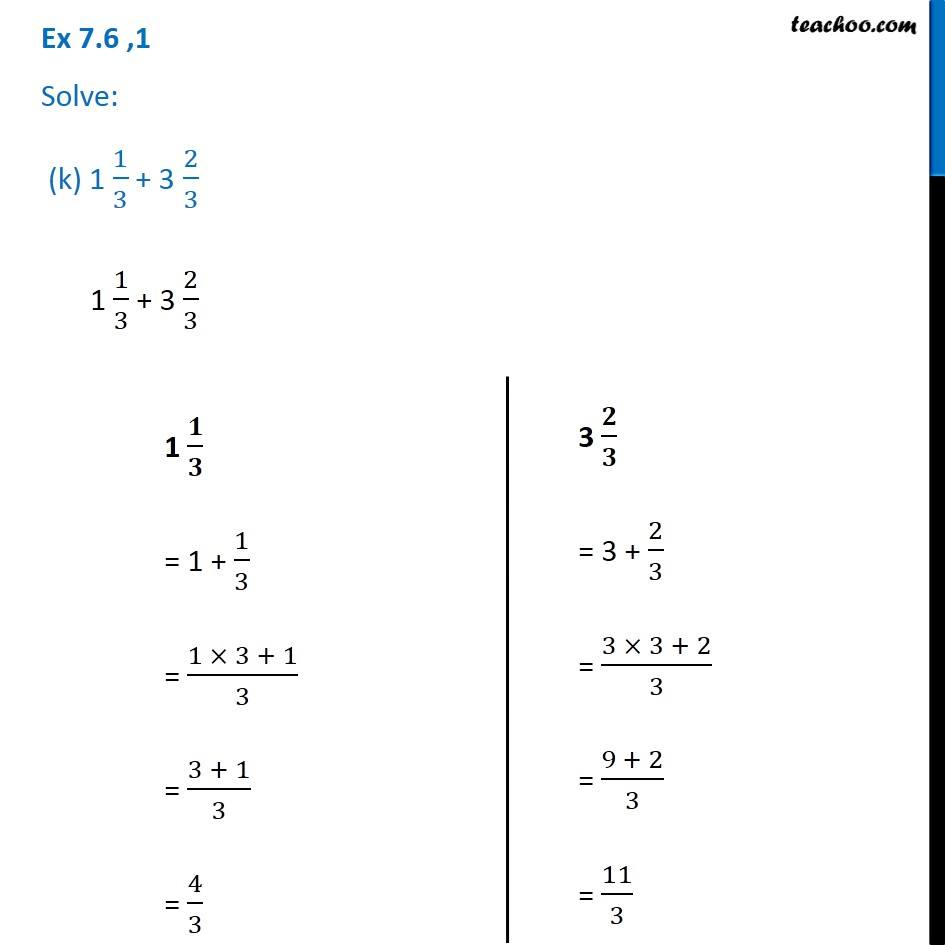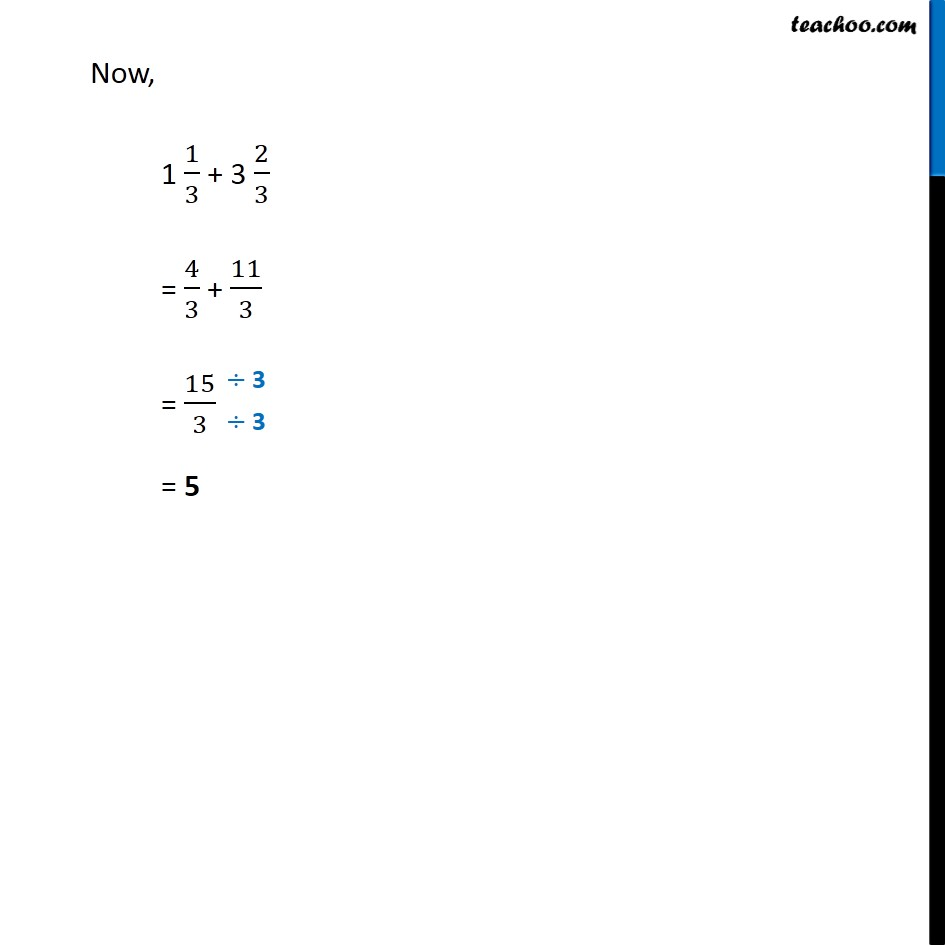1. Chapter 7 Class 6 Fractions
2. Serial order wise
3. Ex 7.6

Transcript

Ex 7.6 ,1 Solve: (k) 1 1/3 + 3 2/3 1 1/3 + 3 2/3 1 / = 1 + 1/3 = (1 3 + 1)/3 = (3 + 1)/3 = 4/3 3 / = 3 + 2/3 = (3 3 + 2)/3 = (9 + 2)/3 = 11/3 Now, 1 1/3 + 3 2/3 = 4/3 + 11/3 = 15/3 = 5